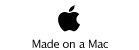JAVA, HTML5 & Chemistry Site

Other Writing

Ad Space for you

Ad Space for you

Last Update
19-Oct-2014

Chemical Engineering: How to get Antoine parameters

2011.4.20

Lecture note of Dr. Hiroshi Yamamoto

## <!-- document.write("<p>Check the browser <BR>"); var userAgent = window.navigator.userAgent.toLowerCase(); var appVersion = window.navigator.appVersion.toLowerCase(); var canrun=0; if (userAgent.indexOf("msie") > -1) { if (appVersion.indexOf("msie 6.0") > -1) { document.write("IE 6.0 does not support HTML5. Please use Chrome, Safari or FireFox. <BR>"); } else if (appVersion.indexOf("msie 7.0") > -1) { document.write("IE 7.0 does not support HTML5. Please use Chrome, Safari or FireFox. <BR>"); } else if (appVersion.indexOf("msie 8.0") > -1) { document.write("IE 8.0 does not support HTML5. Please use Chrome, Safari or FireFox. <BR>"); } else if (appVersion.indexOf("msie 9.0") > -1) { document.write("IE9 may run this simulator but out of support. Please use Chrome, Safari or FireFox. <BR>"); } else { document.write("Unkown Browser. Please use Chrome, Safari or FireFox. <BR>"); } } else if (userAgent.indexOf("firefox") > -1) { canrun=1; } else if (userAgent.indexOf("opera") > -1) { canrun=1; } else if (userAgent.indexOf("chrome") > -1) { canrun=1; } else if (userAgent.indexOf("safari") > -1) { canrun=1; } else { document.write("Unkown Browser. Please use Chrome, Safari or FireFox. <BR>"); } if(canrun==1){ document.write("This Browser will run HTML5. <BR>"); } // -->

HTML5 version (2011.4.20）
JAVA new vwersion 2011.4.21
JAVAold version 2000.1.3

Newest version is implemented into HSPiP ver.4(Y-Predict). If the corporate visitor want to use full version, please buy HSPiP. (2013.1.18)
How to buy HSPiP

### Fitting method

I will explain about fitting method concerning about temperature to vapor pressure relationship to Anotine parameters. With Antoine parameters (A, B, C), vapor pressure at certaine temperature (T) can calculate with this scheme.

log(P[mmHg])=A-B/(T[℃]+C)

The parameters have several variation, log or ln, mmHg or kPa, ℃ or Kelvin, so this parameters are dependent on data books.
Once I know the parameters, then I can calculate vapor pressure at certain temperature with this scheme very easily. I compiled more than 1900 compounds' temperatur dependency of vapor pressur and determined each Antoine prameters. But to tell the truth, these days Antoine equation for vapor pressure fitting is not so popular. I explain the reason.

### 1. Antoine equation can use only limited temperature range.

For example, here is vapor presur-temperature experiment data of n-octanol. If I change the 115℃ data ±1.5mmHg then try to fit Antoine parameters. (temperature 55℃ to 115℃, 17 data points)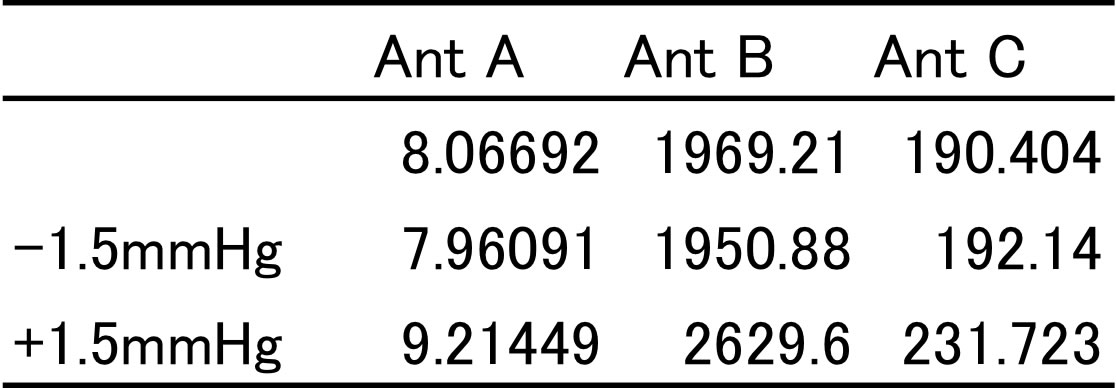If I change upper limit data slightly, the obtained Antoine parameters differ so much. If I calculate vapor pressure at boiling point with these Antoine parameters, the difference of estimated vapor pressure (should be 760mmHg) vary 260mmHg.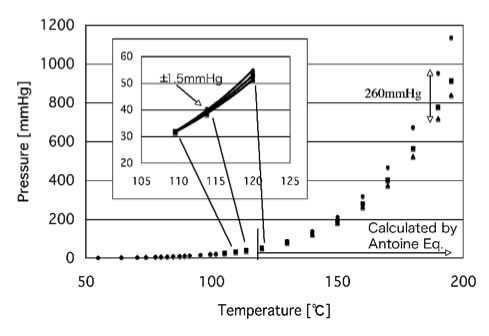So, we need to define temperature range for the Antoine parameters, and it is said there is no expolation ability for this scheme. Why this happen?

I believe this deficit is not problem with Antoine equation but the fitting argorithme. We always use Marquart non-linear least square method to determine these parameters.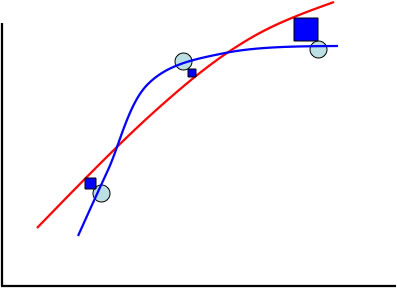I show the problem with above chart.
Suppose we have vapor pressure data marked cyan circle. And we want to get vapor pressure curve showed with red. But the square error from data point to red curve (showed with blue rectangle) become very large at the edge of curve.
The non-linear least square method try to reduce the total rectangle area and answer blue curve. If one want to submit the result into academic paper, the smaller error is much better. So these parameters are widely spread.

### 2. Antoine equation is not accurate, so use more accurate equation.

Extended Antoine Equation
log(P[bar])=A-B/(T[K]+C-273.15)+0.43429Xn+EX6+FX12　 ( X:(T-to-273.15)/Tc )

Wagner Equation
ln Pvpr = (aτ + bτ1.5 + cτ3 + dτ6 )/Tr 　　 (τ=1-Tr)

log P = A + B/T + C*logT+DT+ET2

these day, the third equation become popular. Why they use this equation?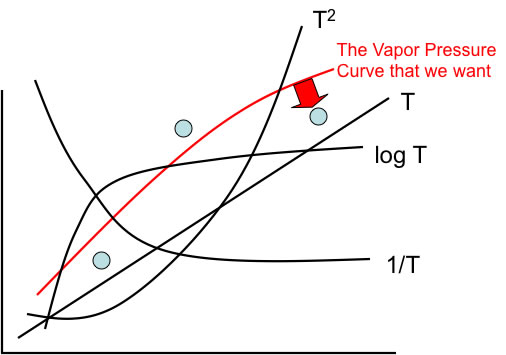This equation make vapor pressure curve with several curve showed above. so if move the edge data slightly to red arrow direction, all of coefficients of curve will change, that reduce total performance. That is mathematically true, but thermo dynamically it become meaningless.

### 3. Antoine equation affected experimental error so much.

When I tried to determine Antoine parameters from vapor pressure experimental data, I got the data like below.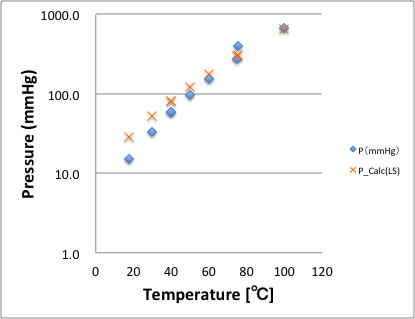This data is Chloroacetyl chloride, the data next to edge of right is definitely abnormal data. And if I plot vapor pressure with the Antoine parameters listed in same data source, the error become large at lower temperature area. The non-linear least square method try to reduce largest error and assign smaller errors to each data point.My program to determine Antoine parameters from vapor pressure Data do not affected by these errors.

### 4. can not determine Antoine parameters from chemical structure.

I've already determined 1900 molecule's Antoine parameter with my method. I show n-alkane result as example. The determined Antoine B parameter by Pririka Method, that are very consistent to Carbon number for both Hydrocarbon molecule and Alcohol compounds. With Marquart Method, determinded Antoine Parameters have no tendency.Antoine C parameter is same tendency with Antoine B Parameter.This result are same for other molecules. Marquart Method determined Antoine parameter so as the total square error become lowest, and never mind the size of molecule. The Group of molecule is same, determined value have some tendency. So after I got 1900 Antoine parameters list, I succeed to build Group Additivity estimation scheme for Antoine Parameter. After I got this scheme, I can get Antoine B, C parameters from only chemical structure. To determine Antoine A parameter, I need boiling point data (or at least one experiment data point). The accurate BP estimation is very hard, so I recommend to use experimental BP, but for user convenience, I put two programs Antoine Parameters. one is Java version and the other is HTML5 version.

### the nature of Antoine Parameters

the basement equation of vapor pressure is Clausius‐Clapeyron equation.

ln(Pvp)=A-B/T  　　B=ΔHv／RΔZv

ΔHv: heat of vaporization, R: Gas constant, ΔZv: difference of compressive factor (very limited range B become constant according to temperature)

So, the B constant in Clausius‐Clapeyron equation, has correlation with Heat of Vaporization. this Clausius‐Clapeyron equation and Antoine equation are so alike, the difference is C parameter.

log(P[mmHg])=A-B/(T[℃]+C)

So, Antoine B parameter also have correlation with Heat of Vaporization. So I tried to check this idea with my database compounds.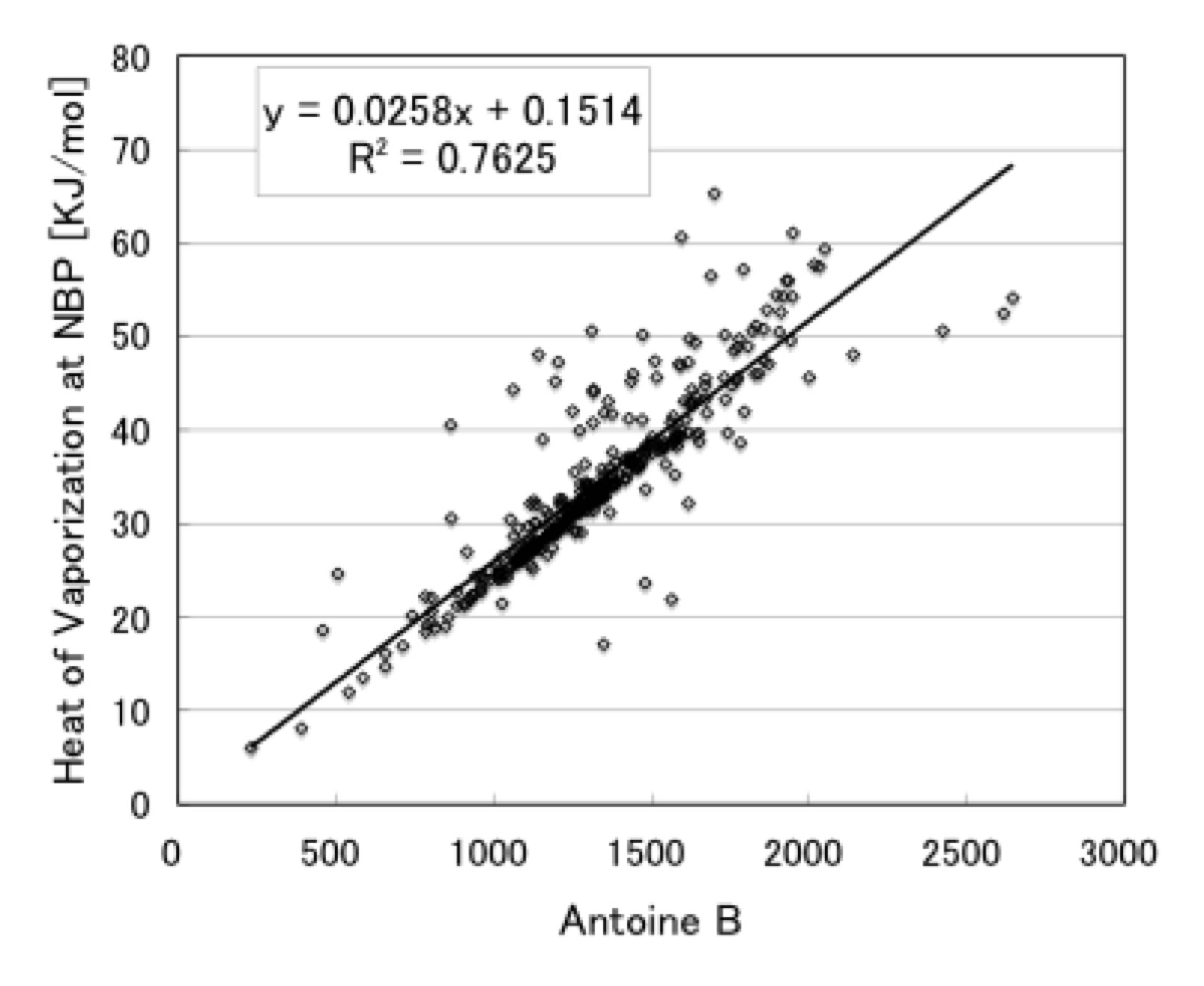the correlation factor is not so high, R2 is just 0.7625.
the reason of this low R2 come from few very large error compounds. Maybe these compounds' Antoine parameters are determined by Marquart Method. But it is very difficult to get Heat of Vaporization from it chemical structure. It is good idea to use Trouton law. this law suggest Hv/BP=21, I plot my database then it become like below.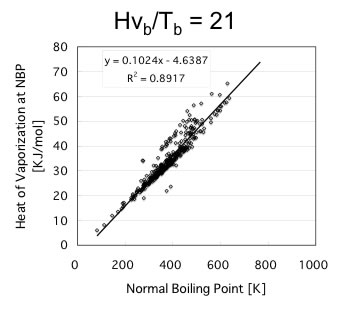So, I can expect Antoine B parameter have correlation with Boiling Point.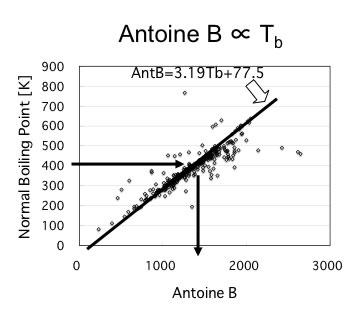I plot the result, and found the Antoine B parameter initial guess can be obtained from boiling point.

Then what Antoine C parameter means?

Kazuo Sato wrote in his book,
Antoine C= 240 - 0.20Tb

Thompson&Fishtine equation
Antoine C= -18 + 0.19*Tb

if I plot my data base, my equation of initial guess of Antoine C is,

Antoine C=291-0.19*Tb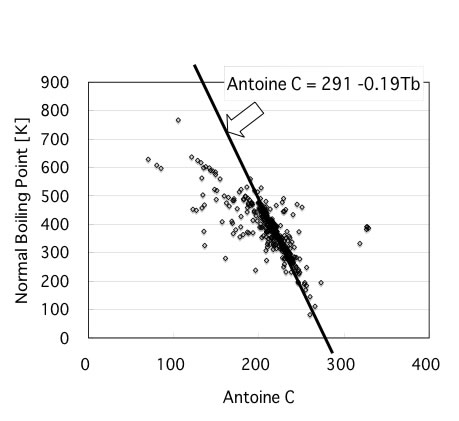The basic idea of Antoine B, C, was published 1923 Cox-Antoine chart I think. I explaine Cox-Antoine chart briefly with alcohol copounds showed below. The vapor pressure - temperature relationship become curves like below.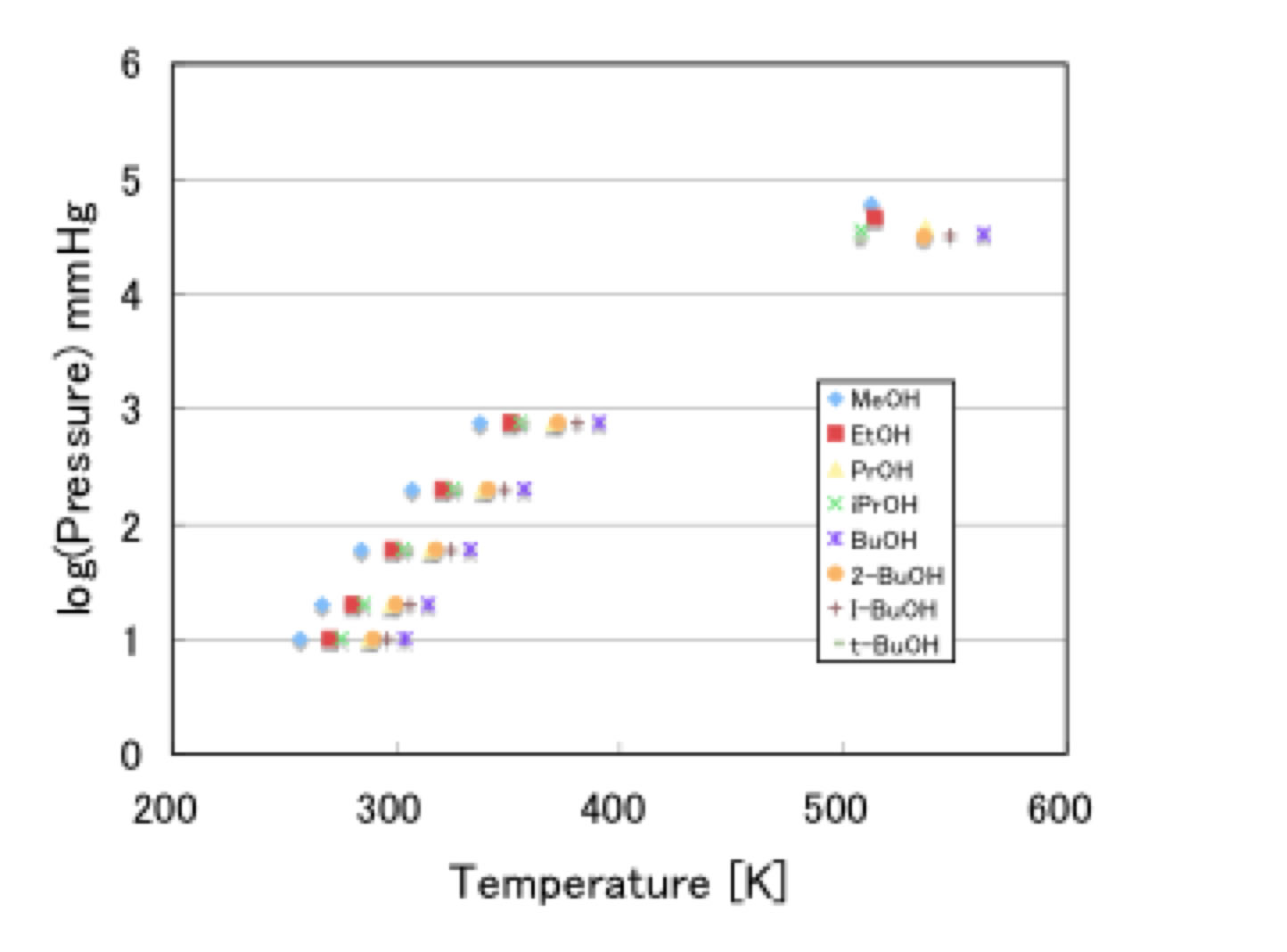And if I use Cox temperature, the curves become lines.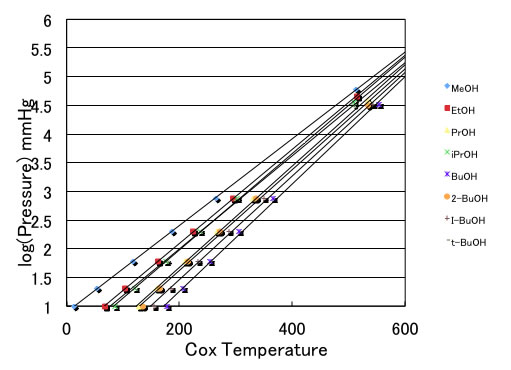And every lines meet at one point called Cox point.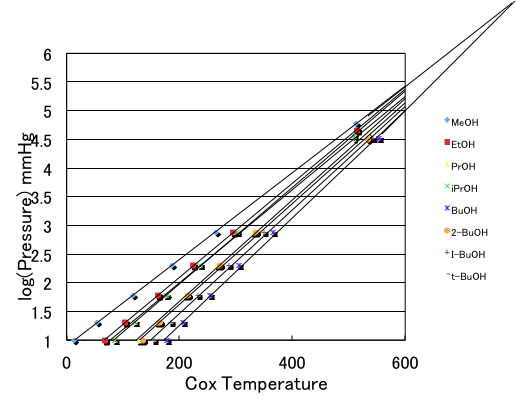So, if we know the Cox point and one vapor pressure data (typically, BP-760mHg), we can get very good vapor pressure - temperature relationship. The Cox point is dependent to type of compounds. please refer to this page.

To determine Cox temperature, they used chart method. For example, water vapor pressure at 293.15K-17.536mmHg, 333.15K-149.44mmHg, 373.15K-760mmH. Then place line on chart, and make Cox temperature. I made Neural Network converter from temperature to Cox temperature.let's see how this Cox temperature work with benzyl alcohol.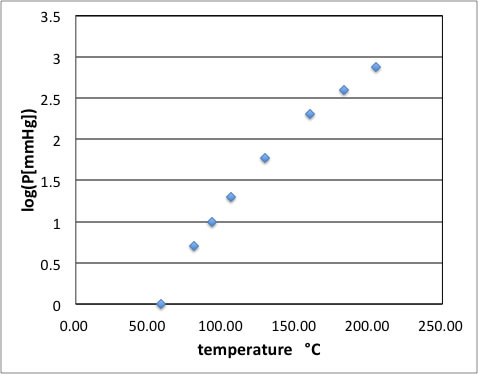This case, vapor pressure - temperature plot become curve.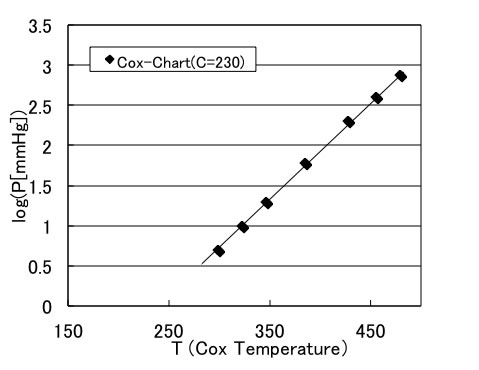If change temperature to Cox temperature, it become line.

If I use Antoine equation C parameter equal 178, it become also line.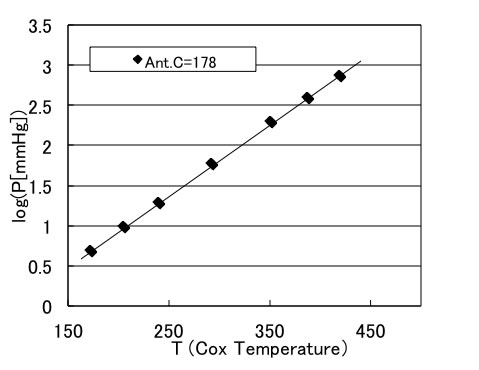So, antoine C erase meander depth, and the Slope is Antoine B.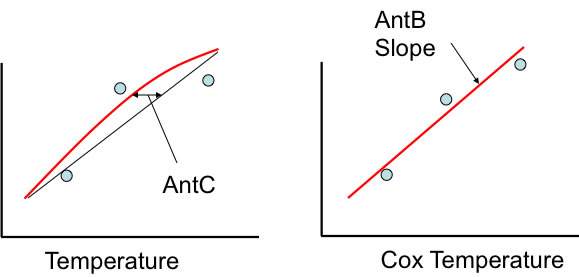This result means that if indivisual type of compounds have one Cox point, then Antoine B and C parameters are predictable from molecular stucture.

So I tried and build the program that estimate Antoine B and C parameter from structure. Please refer to this page if you are interested in this technique.